Latest Banking jobs   »

# Quantitative Aptitude Quiz For NABARD Grade A 2022- 22nd July

Directions (1-5): Please understand the data carefully and answer the following questions.
The table below shows the investment of five different persons, the time for which they invested and rate of interest per annum.
Note:
You have to only calculate the simple interest unless asked for compound interest.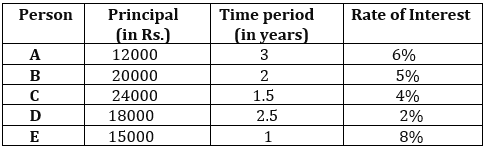Q1. Find the simple interest received by D is what percent less/more than the simple interest received by E?
(a)75%
(b)20%
(c)10%
(d)25%
(e)50%

Q2. Find the difference between the simple interest and compound interest received by B for the same amount at the same rate and time period?
(a) Rs.25
(b) Rs.75
(c)Rs.150
(d)Rs.100
(e)Rs.50

Q3. Find the respective ratio of simple interest received by C to the total amount received by A?
(a)7: 51
(b)5: 51
(c)6: 59
(d)8: 59
(e)3:52

Q4. Find the amount received by B is what more/less than the amount received by D? (in Rs.)
(a)2100
(b)3100
(c)2700
(d)3400
(e)1900

Q5. If interest received by F is 20% of the combined interest of D & E, Moreover, F invested the money for 2 years at 1% more than the rate at which C invested. Find the sum invested by F.
(a)Rs.8400
(b)Rs.9600
(c)Rs.9000
(d)Rs.8000
(e)Rs.9400

Directions (6-10): Please understand the data carefully and answer the following questions.
The following bar graph shows the number of days taken by five different persons individually, P, Q, R, S & T, to finish the work completely.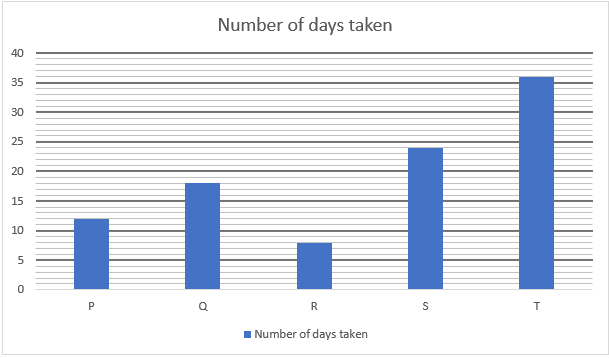Q6. If S and T started doing work together and T left after 6 days, then find the time taken by S to finish the remaining work? (in days)
(a)8
(b)10
(c)16
(d)12
(e)14

Q7. Find the difference between the number of days taken by R & S together and Q & T together to finish the whole work? (in days)
(a)1
(b)12
(c)6
(d)9
(e)3

Q8. If another person, V’s efficiency is 50% more than that of S, then find the time taken by V to complete the work is what percent more/less than the P to finish the work? (in days)
(a)33⅓%
(b)100%
(c)25%
(d)75%
(e)66⅔%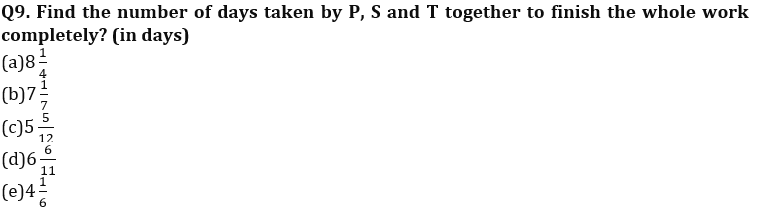Q10. If R and S decide to work together but alternately starting from S, then find the number of days taken to finish the work? (in days)
(a)8
(b)12
(c)16
(d)6
(e)10
Directions (11-15): Please understand the data carefully and answer the following questions.
The following table shows the length (in meters) and speed (in m/s) of five different trains, A, B, C, D & E.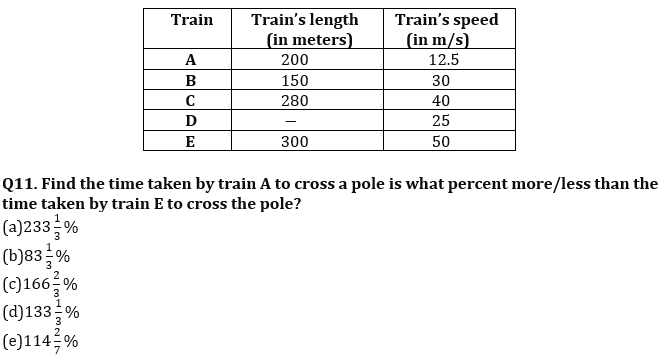Q12. If length of train D is 50% more than the length of train B, then find the respective ratio of time taken by train C to cross a pole to the time taken by train D to cross the pole.
(a)9:8
(b)8:7
(c)7:6
(d)9:5
(e)7:9

Q13. Find the time taken by train A and train E to completely cross each other, if they are moving in opposite direction? (in sec)
(a)10
(b)8
(c)12
(d)6
(e)15

Q14. If train F crosses a pole and platform in 8 seconds and 20 seconds respectively, then find the length of train F, given that length of platform is 240 meters?
(a)160 meter
(b)200 meter
(c)240 meter
(d)120 meter
(e)300 meter

Q15. Find the time taken by train B and train C to completely cross each other, if they are running in same direction? (in sec)
(a)39
(b)55
(c)47
(d)43
(e)51

Solutions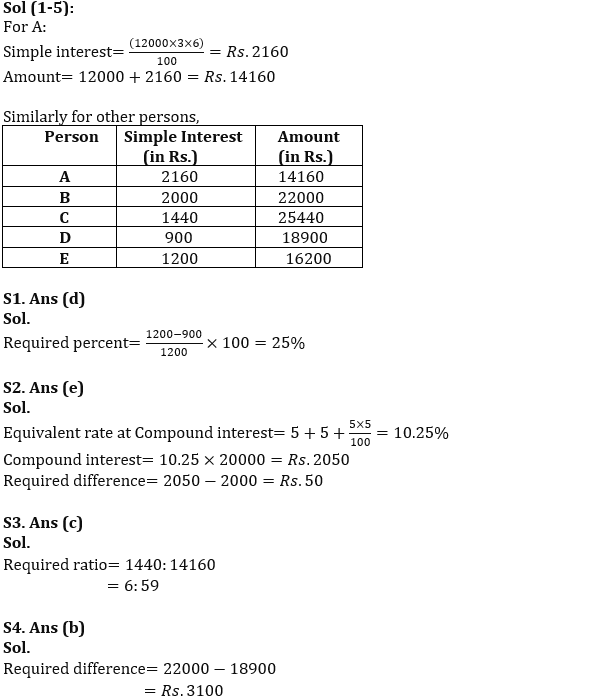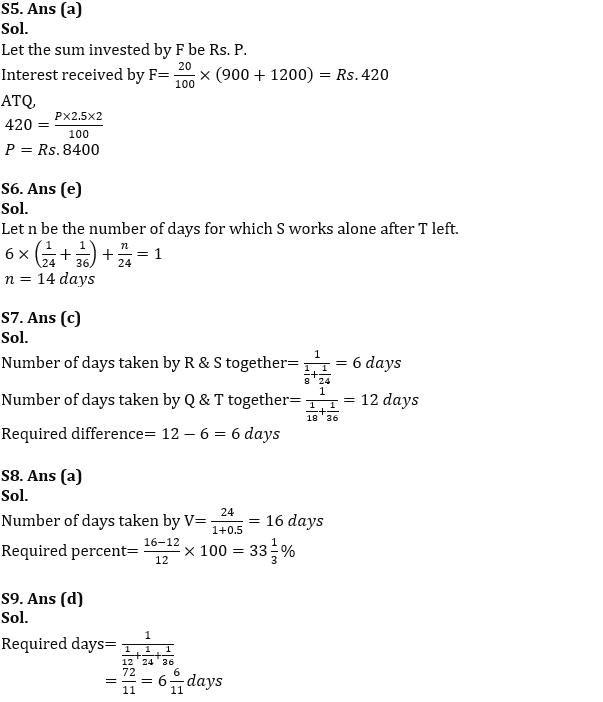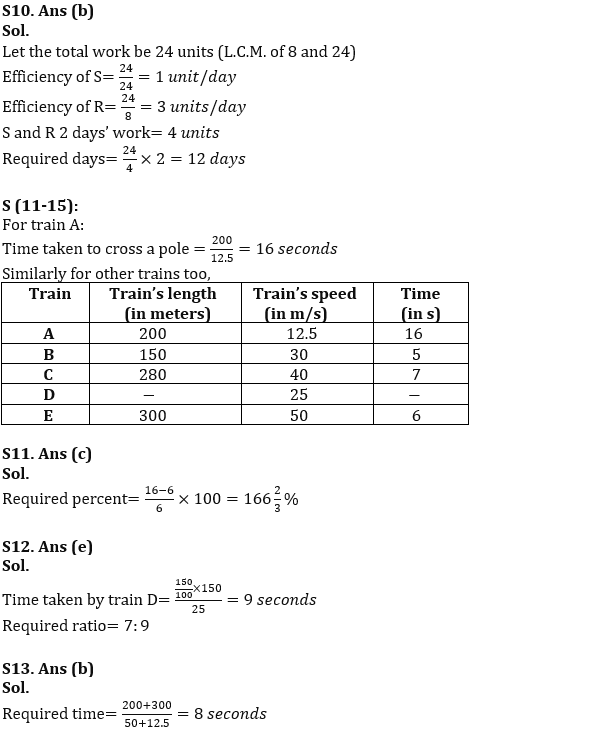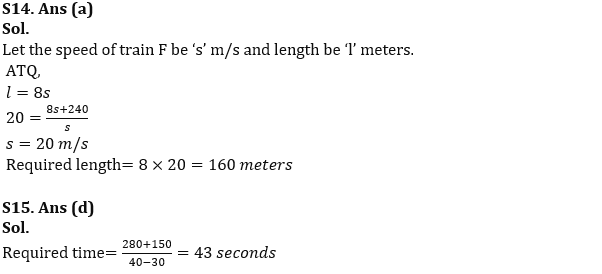#### Congratulations!Incorrect details? Fill the form again here

•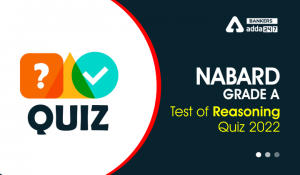Reasoning Ability Quiz For NABARD Grade ...
•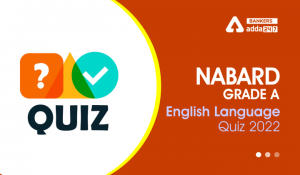English Quizzes For NABARD Grade A 2022-...
•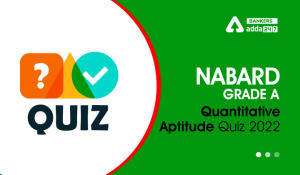Quantitative Aptitude Quiz For NABARD Gr...
•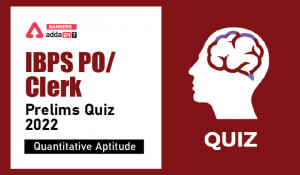Quantitative Aptitude Quiz For IBPS Cler...
•Reasoning Ability Quiz For NABARD Grade ...
•English Quizzes For NABARD Grade A 2022-...